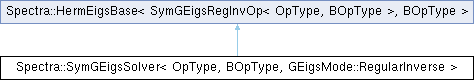Spectra  1.0.1 Header-only C++ Library for Large Scale Eigenvalue Problems
Spectra::SymGEigsSolver< OpType, BOpType, GEigsMode::RegularInverse > Class Template Reference

#include <Spectra/SymGEigsSolver.h>

Inheritance diagram for Spectra::SymGEigsSolver< OpType, BOpType, GEigsMode::RegularInverse >:## Public Member Functions

SymGEigsSolver (OpType &op, BOpType &Bop, Index nev, Index ncv)Public Member Functions inherited from Spectra::SymEigsBase< SymGEigsRegInvOp< OpType, BOpType >, BOpType >
void init (const Scalar *init_resid)

void init ()

Index compute (SortRule selection=SortRule::LargestMagn, Index maxit=1000, Scalar tol=1e-10, SortRule sorting=SortRule::LargestAlge)

CompInfo info () const

Index num_iterations () const

Index num_operations () const

Vector eigenvalues () const

virtual Matrix eigenvectors (Index nvec) const

virtual Matrix eigenvectors () const

## Detailed Description

### template<typename OpType, typename BOpType> class Spectra::SymGEigsSolver< OpType, BOpType, GEigsMode::RegularInverse >

This class implements the generalized eigen solver for real symmetric matrices in the regular inverse mode, i.e., to solve $$Ax=\lambda Bx$$ where $$A$$ is symmetric, and $$B$$ is positive definite with the operations defined below.

This solver requires two matrix operation objects: one for $$A$$ that implements the matrix multiplication $$Av$$, and one for $$B$$ that implements the matrix-vector product $$Bv$$ and the linear equation solving operation $$B^{-1}v$$.

If $$A$$ and $$B$$ are stored as Eigen matrices, then the first operation can be created using the DenseSymMatProd or SparseSymMatProd classes, and the second operation can be created using the SparseRegularInverse class. There is no wrapper class for a dense $$B$$ matrix since in this case the Cholesky mode is always preferred. If the users need to define their own operation classes, then they should implement all the public member functions as in those built-in classes.

Template Parameters
 OpType The name of the matrix operation class for $$A$$. Users could either use the wrapper classes such as DenseSymMatProd and SparseSymMatProd, or define their own that implements the type definition Scalar and all the public member functions as in DenseSymMatProd. BOpType The name of the matrix operation class for $$B$$. Users could either use the wrapper class SparseRegularInverse, or define their own that implements all the public member functions as in SparseRegularInverse. Mode Mode of the generalized eigen solver. In this solver it is Spectra::GEigsMode::RegularInverse.

Definition at line 251 of file SymGEigsSolver.h.

## ◆ SymGEigsSolver()

template<typename OpType , typename BOpType >
 Spectra::SymGEigsSolver< OpType, BOpType, GEigsMode::RegularInverse >::SymGEigsSolver ( OpType & op, BOpType & Bop, Index nev, Index ncv )
inline

Constructor to create a solver object.

Parameters
 op The $$A$$ matrix operation object that implements the matrix-vector multiplication operation of $$A$$: calculating $$Av$$ for any vector $$v$$. Users could either create the object from the wrapper classes such as DenseSymMatProd, or define their own that implements all the public members as in DenseSymMatProd. Bop The $$B$$ matrix operation object that implements the multiplication operation $$Bv$$ and the linear equation solving operation $$B^{-1}v$$ for any vector $$v$$. Users could either create the object from the wrapper class SparseRegularInverse, or define their own that implements all the public member functions as in SparseRegularInverse. $$B$$ needs to be positive definite. nev Number of eigenvalues requested. This should satisfy $$1\le nev \le n-1$$, where $$n$$ is the size of matrix. ncv Parameter that controls the convergence speed of the algorithm. Typically a larger ncv means faster convergence, but it may also result in greater memory use and more matrix operations in each iteration. This parameter must satisfy $$nev < ncv \le n$$, and is advised to take $$ncv \ge 2\cdot nev$$.

Definition at line 283 of file SymGEigsSolver.h.

The documentation for this class was generated from the following file: